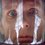# An interesting trigonometric integral

Once while solving problems from Romanian Mathematical Olympiads I encountered a mathematical gem. The problem I found was asking to calcualte the following integral $I=\int\frac{\cos x}{a\sin x+b\cos x}\,dx.$

Solution. Let's try to evaluate one more integral $J=\int{\frac{\sin x}{a\sin x+b\cos x}}\,dx.$

Can you guess the next step?

Yes, we will take advantage of the fact that the sum of integrals is the integral of sum. In other words: $aJ+bI=a\int{\frac{\sin x}{a\sin x+b\cos x}}\,dx+b\int{\frac{\cos x}{a\sin x+b\cos x}}\,dx\\=\int{\frac{a\sin x+b\cos x}{a\sin x+b\cos x}}\,dx=x+C.$

On other hand we can easily calculate integrals of the form $\int{\frac{f^\prime(x)}{f(x)}}\,dx$. The derivative of $(a\sin x+b\cos x)$ is equal to $(a\cos x-b\sin x)$, which can also be expressed as a linear combination of our two integrals, i.e. $aI-bJ=\int{\frac{a\cos x-b\sin x}{a\sin x+b\cos x}}\,dx=\ln{|a\sin x+b\cos x|}+C.$

Now we simply have to solve a system of linear equations. We obtain $I=\frac{a\ln{|a\sin x+b\cos x|}+bx}{a^2+b^2}+C.$ and $J=\frac{ax-\ln{|a\sin x+b\cos x|}}{a^2+b^2}+C.$

Has anyone of you seen problems with the similar ideas? How often while solving a problem you investigate possible variations of the conditions?Note by Nicolae Sapoval
6 years, 9 months ago

This discussion board is a place to discuss our Daily Challenges and the math and science related to those challenges. Explanations are more than just a solution — they should explain the steps and thinking strategies that you used to obtain the solution. Comments should further the discussion of math and science.

When posting on Brilliant:

• Use the emojis to react to an explanation, whether you're congratulating a job well done , or just really confused .
• Ask specific questions about the challenge or the steps in somebody's explanation. Well-posed questions can add a lot to the discussion, but posting "I don't understand!" doesn't help anyone.
• Try to contribute something new to the discussion, whether it is an extension, generalization or other idea related to the challenge.

MarkdownAppears as
*italics* or _italics_ italics
**bold** or __bold__ bold
- bulleted- list
• bulleted
• list
1. numbered2. list
1. numbered
2. list
Note: you must add a full line of space before and after lists for them to show up correctly
paragraph 1paragraph 2

paragraph 1

paragraph 2

[example link](https://brilliant.org)example link
> This is a quote
This is a quote
    # I indented these lines
# 4 spaces, and now they show
# up as a code block.

print "hello world"
# I indented these lines
# 4 spaces, and now they show
# up as a code block.

print "hello world"
MathAppears as
Remember to wrap math in $$ ... $$ or $ ... $ to ensure proper formatting.
2 \times 3 $2 \times 3$
2^{34} $2^{34}$
a_{i-1} $a_{i-1}$
\frac{2}{3} $\frac{2}{3}$
\sqrt{2} $\sqrt{2}$
\sum_{i=1}^3 $\sum_{i=1}^3$
\sin \theta $\sin \theta$
\boxed{123} $\boxed{123}$

Sort by:

Try solving this the same way: $\Large I = \int \frac {a \sin x + b \cos x }{c \sin x + d \cos x} \,dx$

- 6 years, 9 months ago

1. Lets split the integral $I$ into the sum of two integrals: $I=a\int\frac{\sin x}{c\sin x+d\cos x}\,dx+b\int\frac{\cos x}{c\sin x+d\cos x}\,dx=aJ+bK.$
2. Now we reduced our problem to the previous one, because integrals $J,K$ are exactly the same as the one mentioned in the post.

- 6 years, 9 months ago

Smiley_Face.gif

- 6 years, 9 months ago

- 6 years, 4 months ago

This looks very useful, thanks for sharing Nicolae. :)

- 6 years, 9 months ago

Thanks for sharing !!

- 6 years, 9 months ago

- 6 years, 4 months ago

Thanks for sharing

- 6 years, 8 months ago